SHARE
HELP

To start with, Sr2Jr’s first step is to reduce the expenses related to education. To achieve this goal Sr2Jr organized the textbook’s question and answers. Sr2Jr is community based and need your support to fill the question and answers. The question and answers posted will be available free of cost to all.# Absolute C++

Authors:
Walter Savitch ,kenrick Mock
Exercise:
Programming Projects
Chapter:
Arrays
Edition:
5
ISBN:
9780132846813
Question:
1
 Previous Next

###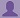Question

Write a program that reads in the average monthly rainfall for a city for each month of the year and then reads in the actual monthly rainfall for each of the previous 12 months. The program then prints out a nicely formatted table showing the rainfall for each of the previous 12 months as well as how much above or below average the rainfall was for each month. The average monthly rainfall is given for the months January, February, and so forth, in order. To obtain the actual rainfall for the previous 12 months, the program first asks what the current month is and then asks for the rainfall figures for the previous 12 months. The output should correctly label the months.

There are a variety of ways to deal with the month names. One straightforward method is to code the months as integers and then do a conversion before doing the output. A large switch statement is acceptable in an output function. The month input can be handled in any manner you wish, as long as it is relatively easy and pleasant for the user.

After you have completed the previous program, produce an enhanced version that also outputs a graph showing the average rainfall and the actual rainfall for each of the previous 12 months. The graph should be similar to the one shown in Display 5.4, except that there should be two bar graphs for each month and they should be labeled as the average rainfall and the rainfall for the most recent month. Your program should ask the user whether he or she wants to see the table or the bar graph, and then should display whichever format is requested. Include a loop that allows the user to see either format as often as the user wishes until the user requests that the program end.

###Answer

Program:

``````#include <iostream>
#include <string>
#include <cmath>
#include <fstream>
#include <cstdlib>
using namespace std;
void monthNames(string []);
void getInputFile(ifstream&, const char*);
void getOutputFile(ofstream&, const char*);
double average(double, double);
string displayDots(double);
void displayChart(ofstream&, string [], double [], double []);
void displayGraph(ofstream&, string [], double [], double []);
const char currentFile = "rainfallCurrent.dat";
const char previousFile = "rainfallPrevious.dat";
const char outputFile = "rainfallOutput.dat";
const int SIZEMONTH = 12;
int main()
{
ifstream currentRainfall, prevRainfall;
ofstream resultRainfall;
double current[SIZEMONTH], previous[SIZEMONTH];
getInputFile(currentRainfall, currentFile);
getInputFile(prevRainfall, previousFile);
getOutputFile(resultRainfall, outputFile);
string months[SIZEMONTH];
monthNames(months);
displayChart(resultRainfall, months, current, previous);
displayGraph(resultRainfall, months, current, previous);
resultRainfall.close();
return 0;
}
double average(double current, double previous)
{
double out = (current+previous)/2;
double avg = out;
if(out < current) {
avg *= -1;
}
return avg;
}
string displayDots(double x)
{
string dots = "*";
for(double i = 0; i < x;i+=0.1) {
dots += "*";
}
return dots;
}
void displayGraph(ofstream& file, string month[], double current[] , double previous[])
{
file << "--------------------" << endl;
for(int i = 0; i < SIZEMONTH;i++) {
file << month[i] << " - GRAPH" << endl;
file << "--------------------" << endl;
file << " Current Year: " << displayDots(current[i]) << endl;
file << "      Average: " << displayDots(average(current[i], previous[i])) << endl;
file << "--------------------" << endl;
}
file << endl;
}
void displayChart(ofstream& file, string month[], double current[] , double previous[]) {
file.setf(ios::fixed);
file.setf(ios::showpoint);
file.precision(2);
file << "--------------------" << endl;
for(int i = 0; i < SIZEMONTH;i++) {
file << month[i] << " - CHART"  << endl;
file << "--------------------" << endl;
file << " Current Year:  " << current[i] << endl;
file << "Previous Year:  " << previous[i] << endl;
file.setf(ios::showpos);
file << "      Average: " << average(current[i], previous[i]) << endl;
file.unsetf(ios::showpos);
file << "--------------------" << endl;
}
file << endl;
}
void monthNames(string mm[])
{
mm = "January";
mm = "February";
mm = "March";
mm = "April";
mm = "May";
mm = "June";
mm = "July";
mm = "August";
mm = "September";
mm = "October";
mm = "November";
mm = "December";
}
{
double d;
for(int i = 0;i < SIZEMONTH;i++)
{
file >> d;
month[i] = d;
}
}

void getOutputFile(ofstream& file, const char* fileName)
{
file.open(fileName);
if (file.fail())
{
cout << "Error: " << fileName << " can't be opened." << endl;
exit(1);
}
cout << "NOTICE: " << fileName << " opened successfully." << endl;
}

void getInputFile(ifstream& file, const char* fileName)
{
file.open(fileName);
if (file.fail())
{
cout << "Error: " << fileName << " can't be opened." << endl;
exit(1);
}
cout << "NOTICE: " << fileName << " opened successfully." << endl;
}       ``````

Input files data:

rainfallCurrent.dat:

11
9
6
10
1.5
2.5
5.5
14
3.5
2.5
3.5
3
rainfallPrevious.dat:
10.5
9.3
5.5
1
0
0.5
8
11
12.5
11.7
4.
3.6
Output:

rainfallOutput.dat: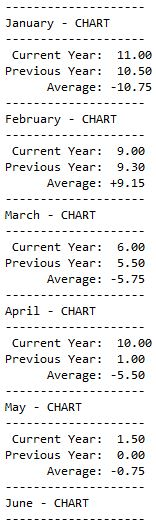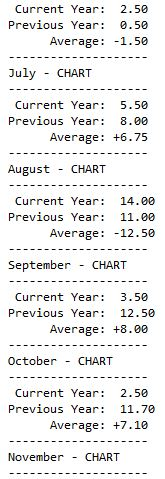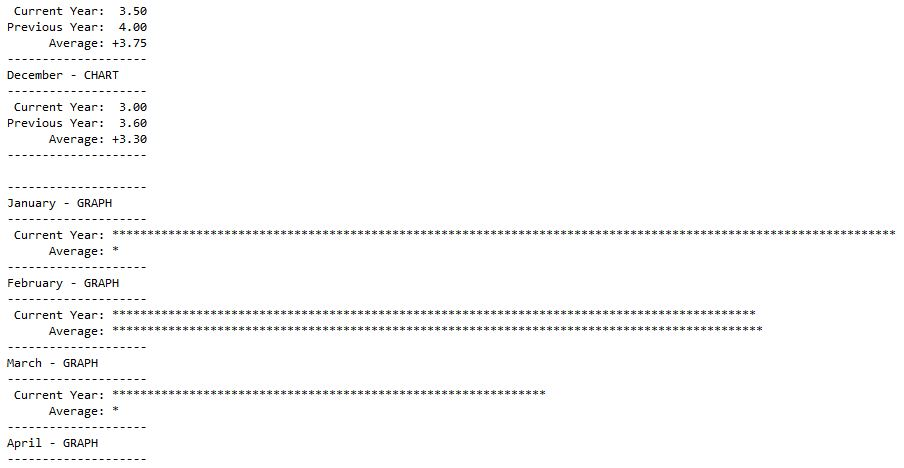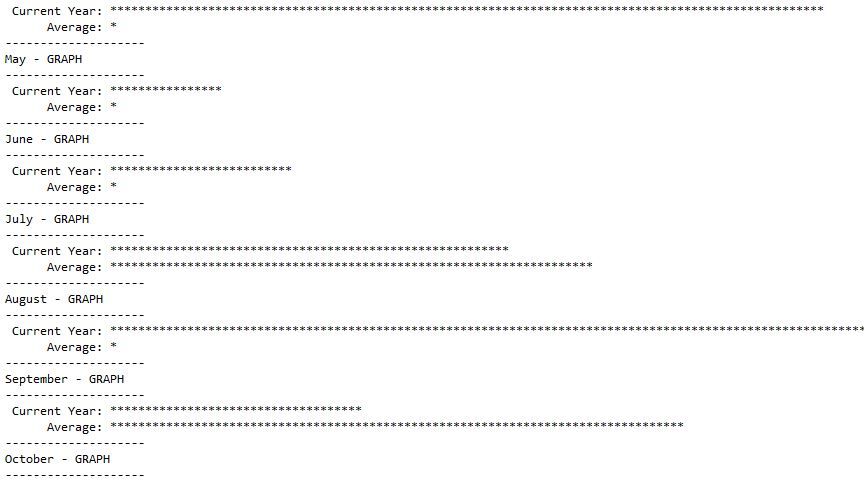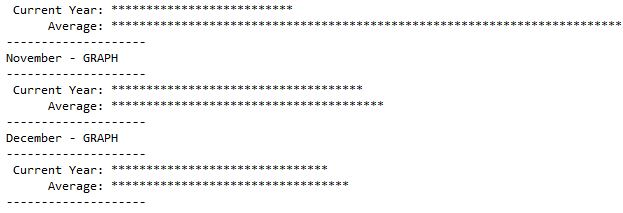### Discussions

Post the discussion to improve the above solution.